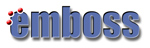NUCLEIC COMPOSITION

Composition of nucleotide sequences

Program nameDescription
banana Plot bending and curvature data for B-DNA
btwisted Calculate the twisting in a B-DNA sequence
chaos Draw a chaos game representation plot for a nucleotide sequence
compseq Calculate the composition of unique words in sequences
dan Calculates nucleic acid melting temperature
density Draw a nucleic acid density plot
freak Generate residue/base frequency table or plot
isochore Plots isochores in DNA sequences
wordcount Count and extract unique words in molecular sequence(s)